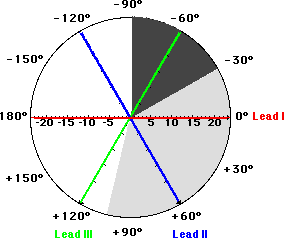slideshow widget

## Wednesday, November 3, 2010

### The basics of Axis Deviation of the EKGIn this post you'll learn about axis deviation of the EKG, and how you can use it to determine the underlying pathway of the patient.

Axis deviation is the general direction the electrical signal takes from the AV nodes through the ventricles. The direction of depolarization (vector) and size of the QRS arrow (amplitude) change with certain disease processes.

If the axis is not depolarizing normally, this can help clue you in to underlying pathology.

So, to determine axis, check out the hexaxial reference. Examine the QRS complex in the limb leads (I, II and III). Now find the one that has the largest deflection (amplitude). Now determine if it's upright (positive) or downward (negative).

If the largest amplitude is lead II, and the amplitude is positive, then you know you have an axis deviation of about +60, which is normal. If, on the other hand, the amplitude was negative, then the deviation is about -120, which is severe right axis deviation.

Basically, if the QRS is upright (positive) in the lead with the most voltage, the mean axis must be very close to the position of this lead on the circle. If the QRS complex is downward (negative), the mean axis must be located in the opposite direction from the location of this lead on the hexaxial circle.

It's that simple.

To make it even easier (we RTs love to keep things simple), if you look at the standard EKG, the axis will be noted for you, so you shouldn't even have to use this hexaxial reference.

Now determine axis (again, this should be noted on the EKG):

• Normal axis is 0 to +90 degrees.
• Left Axis Deviation is anything between 0 and -90
• Right Axis Deviation is between +90 and 180
• Severe Right Axis Deviation (no man's land) is anything less than -90

22. Right Axis Deviation: (axis between 9 and -90)

• a. QRS negative in lead I
• b. QRS positive in AVF
• c. QRS negative in AVF and lead I if extreme RAD
• d. QRS in V1, V2 isoelectric
• e. Slender person with ventricular heart
• f. Ventricular hypertrophy
• g. Pulmonary disease
• h. MI on left side of heart
• i. Q-wave of inferior MI
• j. Pulmonary embolism
• k. Anteriolateral MI
• l. Emphysema

23. Left Axis Deviation: (axis between +90 and 180)

• a. QRS positive in lead I
• b. QRS negative in AVF
• c. QRS in V5 and V6 are isoelectric
• d. Obese patients
• e. Left Ventricular Hypertrophy
• f. MI right side of heart

24. Severe Right Axis Deviation: (Axis between -90 and -179)

• Called no mans land
• Severe Left Axis Deviation
• Emphysema